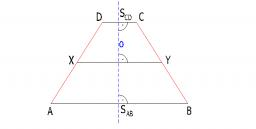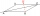# Trapezoid

How long are the trapezoid bases with area 24 cm2 and height 3 cm. One base is 3 times longer than the shorter.

a =  12 cm
c =  4 cm

### Step-by-step explanation:

(a+c)/2•3 = 24
a = 3c

(a+c)/2•3 = 24
a = 3•c

3a+3c = 48
a-3c = 0

a = 12
c = 4

Our linear equations calculator calculates it.Did you find an error or inaccuracy? Feel free to write us. Thank you!Tips to related online calculators
Do you have a linear equation or system of equations and looking for its solution? Or do you have a quadratic equation?

#### You need to know the following knowledge to solve this word math problem:

We encourage you to watch this tutorial video on this math problem:

## Related math problems and questions:

• Dimensions of the trapezoidOne of the bases of the trapezoid is one-fifth larger than its height, the second base is 1 cm larger than its height. Find the dimensions of the trapezoid if its area is 115 cm2
• Isosceles trapezoidCalculate the content of an isosceles trapezoid whose bases are at ratio 5:3, the arm is 6cm long and it is 4cm high.
• Isosceles trapezoidFind the height in an isosceles trapezoid if the area is 520 cm2 and the base a = 25 cm and c = 14 cm. Calculate the interior angles of the trapezoid.
• The basesThe bases of the isosceles trapezoid ABCD have lengths of 10 cm and 6 cm. Its arms form an angle α = 50˚ with a longer base. Calculate the circumference and content of the ABCD trapezoid.
• Isosceles trapezoidFind the area of an isosceles trapezoid, if the bases are 12 cm and 20 cm, the length of the arm is 16 cm
• ISO trapezoid v2bases of Isosceles trapezoid measured 20 cm and 4 cm and its perimeter is 55 cm. What is the are of a trapezoid?
• Trapezoid MO-5-Z8Trapezoid KLMN has bases 12 and 4 cm long. The area of triangle KMN is 9 cm2. What is the area of the trapezoid KLMN?
• KLMN trapezoidThe KLMN trapezoid has bases KL 40cm and MN 16cm. On the KL base is point P. The segment NP divides the trapezoid into units with the same area. What is the distance of point P from point K?
• ParallelogramRhomboid (parallelogram) has a longer side of 50 cm long. The size of its one height is four times the size of its second height. Calculate the length of the shorter side of this rhomboid in the centimeters.
• IS trapezoidCalculate the length of diagonal u and height v of isosceles trapezoid ABCD, whose bases have lengths a = |AB| = 37 cm, c = |CD| = 29 cm and legs b = d = |BC| = |AD| = 28 cm.
• Trapezoidtrapezoid ABCD a = 35 m, b=28 m c = 11 m and d = 14 m. How to calculate its area?The quadrangular prism has a volume of 648 cm3. Trapezoid which is its base has the dimensions bases: a = 10 cm, c = 5 and height v = 6 cm. What is the height of the prism?Rectangular trapezium has bases 21 and 10 and area 92 cm2. What is its perimeter?Find the trapezium height if a = 8 cm and c = 4 cm if its content 21 square centimeters.The isosceles triangle has a circumference of 46 cm. Calculate its area if the arm is 5 cm longer than the base.Trapezoid ABCD has a length of bases in ratio 3:10. The area of triangle ACD is 825 dm2. What is the area of trapezoid ABCD?A trapezoid ABCD has the bases length of a = 120 mm, c = 86 mm and the area A = 2,575 mm2. Find the height of the trapezoid.